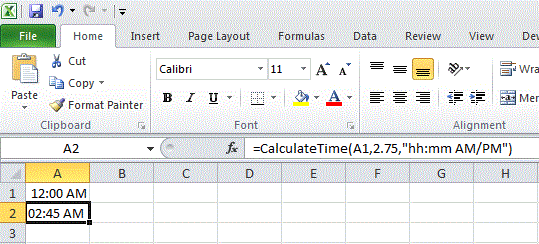## October 31, 2010

### Calculate Time

Adding or subtracting days to a date in Excel is easy but, unless I am missing something, adding hours to a time is a bit harder. Yes, you could use inbuilt functions TIME, with HOUR, MINUTE and SECOND to do it but it's still not the same if you just want to add time in decimals, eg the original time + 2.75 hours. You have to convert the decimal part to minutes and even though it's a simple enough formula to write, it is a bit unwieldy. So I decided to make a custom function that would do the job.

Here is the code,

Function CalculateTime(dOriginTime As Date, dbChangeTime As Double, Optional sFormat As Variant)
Dim iHour As Integer
Dim iMinute As Integer
Dim iChangeHour As Integer
Dim dbChangeMinute As Double

iHour = Hour(dOriginTime)
iMinute = Minute(dOriginTime)
iChangeHour = Int(dbChangeTime)
dbChangeMinute = dbChangeTime - Int(dbChangeTime)
dbChangeMinute = dbChangeMinute * 60

CalculateTime = TimeSerial(iHour + iChangeHour, iMinute + dbChangeMinute, 0)

If Not IsMissing(sFormat) Then
CalculateTime = Format(CalculateTime, sFormat)
End If

End Function

Here is how it works,

My original time is in cell A1. I want to add 2.75 hours (I'm not concerned with the seconds), and I decide to add an optional format of "hh:mm AM/PM" (don't include this argument if you want to use NumberFormat instead)

So my formula is written as,

=CalculateTime(A1,2.75,"hh:mm AM/PM")

And here is how it looks,Hope it comes in handy.

Posted by andrewe at 11:12